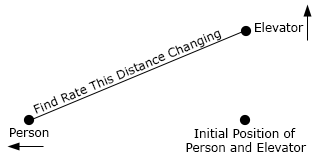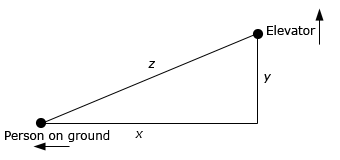Paul's Online Notes
Home / Calculus I / Derivatives / Related Rates
Show Mobile Notice Show All Notes Hide All Notes
Mobile Notice
You appear to be on a device with a "narrow" screen width (i.e. you are probably on a mobile phone). Due to the nature of the mathematics on this site it is best views in landscape mode. If your device is not in landscape mode many of the equations will run off the side of your device (should be able to scroll to see them) and some of the menu items will be cut off due to the narrow screen width.

### Section 3.11 : Related Rates

7. Two people are at an elevator. At the same time one person starts to walk away from the elevator at a rate of 2 ft/sec and the other person starts going up in the elevator at a rate of 7 ft/sec. What rate is the distance between the two people changing 15 seconds later?Show All Steps Hide All Steps

Start Solution

Here is a sketch for this part.We want to determine $$z'$$ after 15 seconds given that $$x' = 2$$, $$y' = 7$$ and assuming that they start at the same point.

Show Step 2

Hopefully it’s clear that we’ll need the Pythagorean Theorem to solve this problem so here is that.

${z^2} = {x^2} + {y^2}$ Show Step 3

Finally, let’s differentiate this with respect to $$t$$ and we can even solve it for $$z'$$ so the actual solution will be quick and simple to find.

$2z\,z' = 2x\,x' + 2y\,y'\hspace{0.5in} \Rightarrow \hspace{0.5in}z' = \frac{{x\,x' + y\,y'}}{z}$ Show Step 4

To finish off this problem all we need to do is determine all three lengths of the triangle in the sketch above. We can find $$x$$ and $$y$$ using their speeds and time while we can find $$z$$ by reusing the Pythagorean Theorem.

$\begin{array}{c}x = \left( 2 \right)\left( {15} \right) = 30\hspace{1.0in}y = \left( 7 \right)\left( {15} \right) = 105\\ z = \sqrt {{{30}^2} + {{105}^2}} = \sqrt {11925} = 15\sqrt {53} = 109.2016\end{array}$

The rate of change of the distance between the two people is then,

$z' = \frac{{\left( {30} \right)\left( 2 \right) + \left( {105} \right)\left( 7 \right)}}{{109.2016}} = \require{bbox} \bbox[2pt,border:1px solid black]{{7.2801}}$